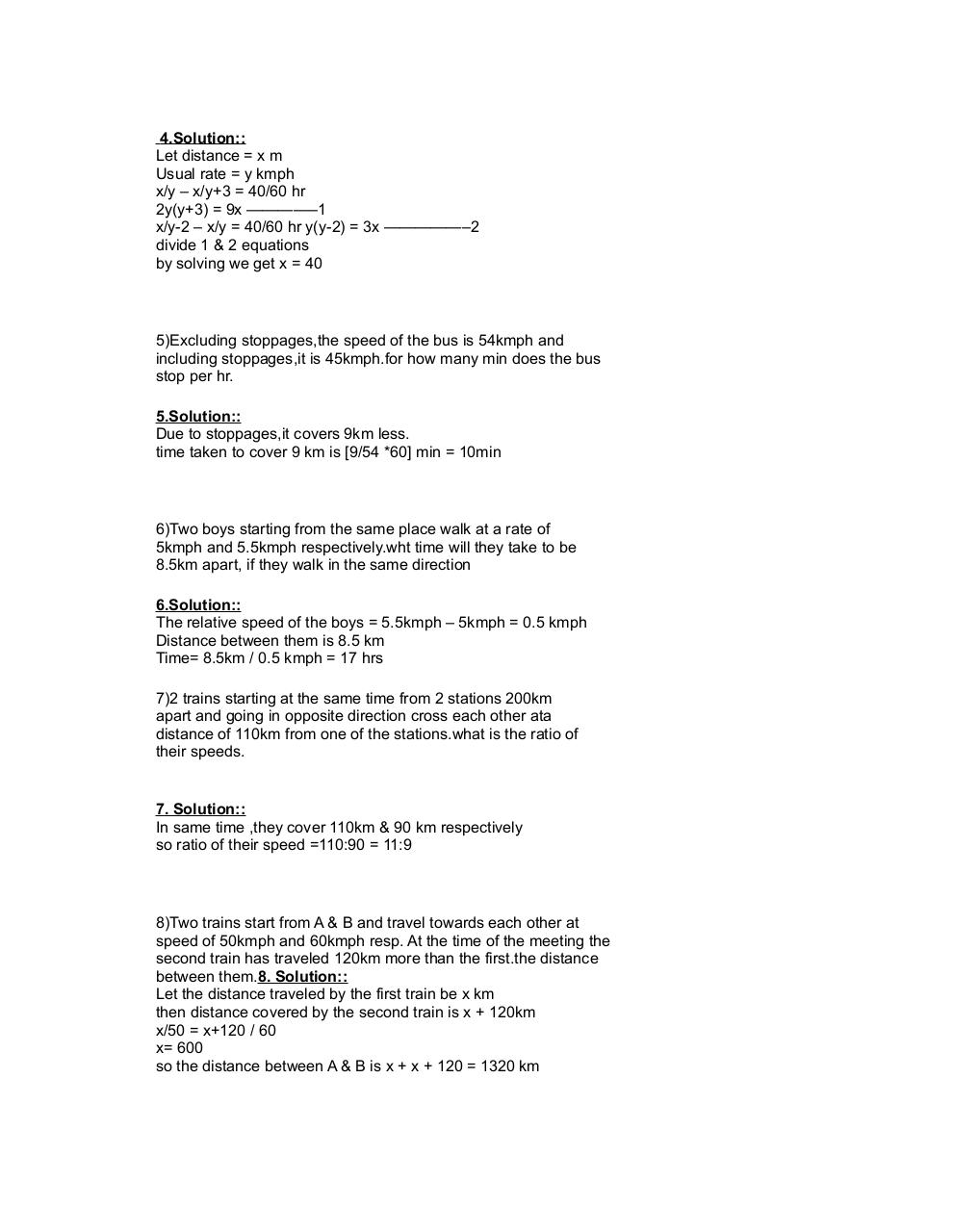# PDF Archive

Easily share your PDF documents with your contacts, on the Web and Social Networks.Page 1 23472

#### Text preview

4.Solution::
Let distance = x m
Usual rate = y kmph
x/y – x/y+3 = 40/60 hr
2y(y+3) = 9x ————–1
x/y-2 – x/y = 40/60 hr y(y-2) = 3x —————–2
divide 1 &amp; 2 equations
by solving we get x = 40

5)Excluding stoppages,the speed of the bus is 54kmph and
including stoppages,it is 45kmph.for how many min does the bus
stop per hr.
5.Solution::
Due to stoppages,it covers 9km less.
time taken to cover 9 km is [9/54 *60] min = 10min

6)Two boys starting from the same place walk at a rate of
5kmph and 5.5kmph respectively.wht time will they take to be
8.5km apart, if they walk in the same direction
6.Solution::
The relative speed of the boys = 5.5kmph – 5kmph = 0.5 kmph
Distance between them is 8.5 km
Time= 8.5km / 0.5 kmph = 17 hrs
7)2 trains starting at the same time from 2 stations 200km
apart and going in opposite direction cross each other ata
distance of 110km from one of the stations.what is the ratio of
their speeds.

7. Solution::
In same time ,they cover 110km &amp; 90 km respectively
so ratio of their speed =110:90 = 11:9

8)Two trains start from A &amp; B and travel towards each other at
speed of 50kmph and 60kmph resp. At the time of the meeting the
second train has traveled 120km more than the first.the distance
between them.8. Solution::
Let the distance traveled by the first train be x km
then distance covered by the second train is x + 120km
x/50 = x+120 / 60
x= 600
so the distance between A &amp; B is x + x + 120 = 1320 km